﻿MESASine Wave

# MESASine Wave

The MesaSinewave utilizes two sine plots to depict whether the market is in a trend mode or in a cycle mode.  If the plots start to wander the market, it is called a trend mode.  The market is in a cyclical mode if the two plots look like a Sine wave.  In a trend mode, the Sine and Lead Sine plots typically weaken in a sideways pattern around the zero-point, running distant and parallel from each other.

MesaSinewave indicator is that it will anticipate cycle mode turning points rather than waiting for confirmation (as the majority of oscillators do).  This may be an extremely helpful trait.  The indicator also has an advantage that wrong signals are minimized in a trend mode.

The indicator contains two plots - one line depicting the Sine of the calculated phase angle over time, and the other the Sine of the phase angle advanced by 45 degrees - the Lead Sine.  The crossings of the Sine and Lead Sine together provide a precise and advanced picture of cycle mode turning points.Market Signals

If the Sine plot crosses below the Lead Sine plot, sell signal is generated.  Buy signal is generated as the Sine plot crosses over the Lead Sine plot if the market is in cycle mode.  It may be worthwhile to trade the trend if the market is in the trend mode.  Main moving average crossovers are often helpful for exiting and entering positions in such markets.Calculation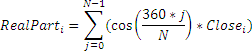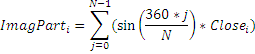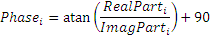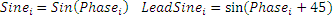where N is a period for indicator calculation.Chart Example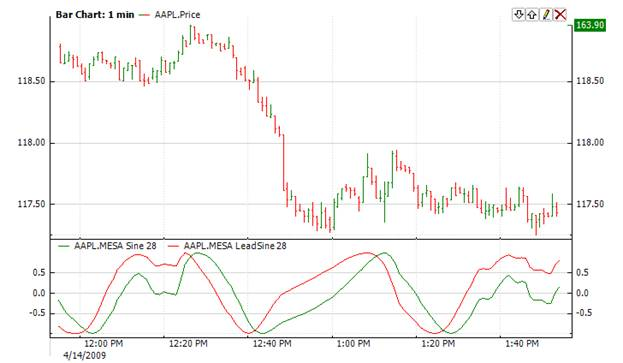Implementation and Usage

To initialize MesaSinewave Index use one of the following constructors:

MesaSinewave – sets default values: period = 14

MesaSinewave(Int32) – sets value for period

Use

Sine

properties to get current valueExample
C#
``` 1// Create new instance
2MesaSinewave mesa = new MesaSinewave(28);
3
4// Number of stored values
5mesa.HistoryCapacity = 2;
6